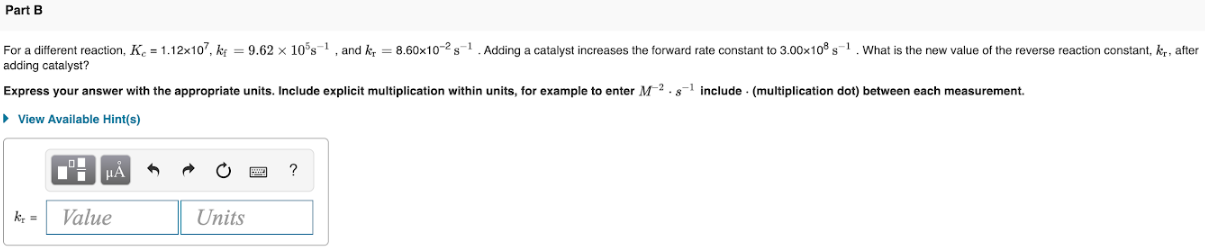# For a different reaction , Kc = 1.12x10^7, kf = 9.62 x 10^5s^-1, and kr = 8.60x10^-2 s^ -1. Adding a catalyst increases the forward rate constant to 3.00x10^8s^-1. What is the new value of the reverse reaction constant, kr, after adding catalyst? Express your answer with the appropriate units. Include explicit multiplication within units, for example to enter M^-2 . s^-1 include. (multiplication dot) between each measurement.